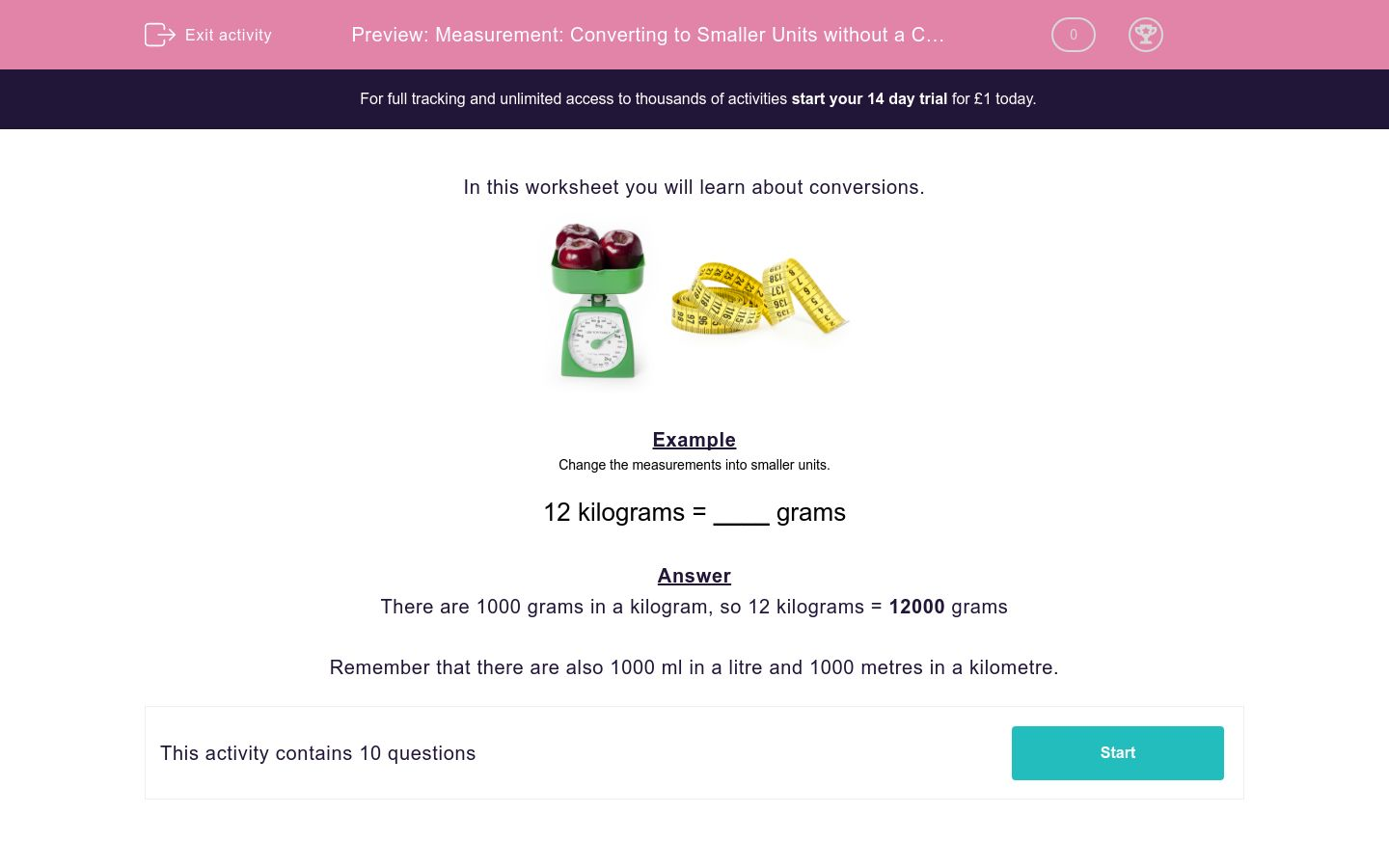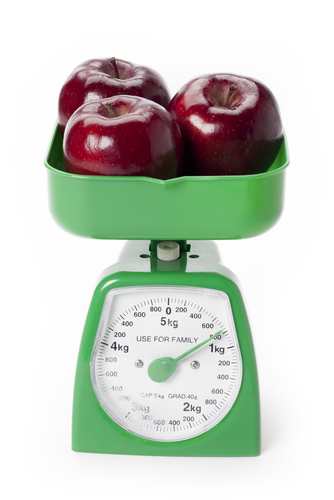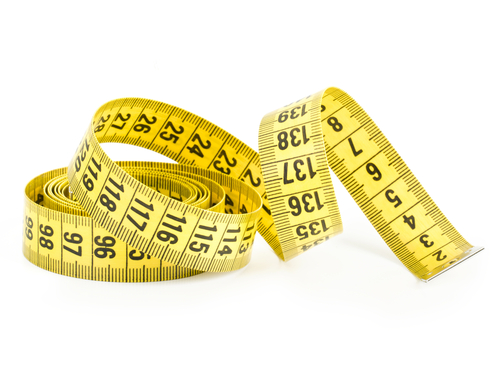# Measurement: Converting to Smaller Units without a Chart

In this worksheet, students convert measurements into smaller units by multiplying by 1000.Key stage:  KS 2

Curriculum topic:   Maths and Numerical Reasoning

Difficulty level:### QUESTION 1 of 10

In this worksheet you will learn about conversions.Example

Change the measurements into smaller units.

12 kilograms = ____ grams

There are 1000 grams in a kilogram, so 12 kilograms = 12000 grams

Remember that there are also 1000 ml in a litre and 1000 metres in a kilometre.

---- OR ----